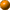# Java ToolsFluorescence pressure calculator. (T<400 K) Calculates pressure according to the shift of the ruby R1 fluorescence line (hydrostatic and non-hydrostatic scales) and Sm-doped SrB4O7 fluorescence line. For T<400 K.Fluorescencepressure calculator. Calculates pressure according to the shift of the ruby R1 fluorescence line (hydrostatic and non-hydrostatic scales) and Sm-doped SrB4O7 fluorescence line. For all temperatures.X-ray diffractionpressure calculator. Calculates pressure according to the equation of state of 16 pressure calibrants from the reduced volume, d-spacing, energy or angle of any given Bragg reflexion.Pressure standard XRD simulator. Calculates the d-spacings and angle or energy positions of the Bragg reflections of 16 pressure standards (metals, salts and oxides) at a given pressure.X-ray diffractionpattern simulator. Calculates the d-spacings and angle or energy dispersive positions of the Bragg reflections of any crystal at a given pressure using an implemented equation of state (Vinet, Birch-Murnaghan, Murnaghan).

Maintained by James Badro